limits

New member
Hello, I have been struggling with this problem and got this far, but I am not sure how to proceed in actually solving to show that f(x) = 0
Any help would be much appreciated.

Consider the function:
$f(x)=(1-1/4x)exp(-x^2+5x-6)$Show the following holds: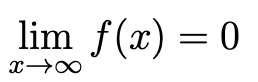I have calculated that
$f'(x)=((2x^2-13x+19)exp(-x^2+5x-6))/4$and from that, that if f(x) = 0, $x=(13+sqrt17)/4$or $x=(13-sqrt17)/4$
but am unsure how to show that this means that x tends to infinity

Jomo

Elite Member
If you want to find when f(x) = 0, why are you computing the derivative?
If you meant you want to solve for f'(x)=0, then why are you trying to do this?
Have you learned L'Hopital's rule? Try it.

New member
that would make a lot more sense thank you!

for L'Hopital's rule would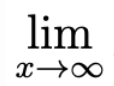$(1-1/4x)exp(-x^2+5x-6)$ would then equal$((2x^2-13x+19)exp(-x^2+5x-6))/4$
or have I made an error since its not f(x)/g(x) and f'(x)/g'(x)

New member
Scratching that, I think I have it worked out.

Instead, I changed $exp(-x^2+5x-6)$ to $exp(x^2+5x+6)$, putting it as the denominator of the fraction with $(1-x/4)$ on top.

I then differentiated them separately once, and then again to get
$0/(4x^2-20x+27)exp(x^2-5x+6)$ which is equal to 0.

Does this answer the question? I'm sorry I have never used the L'Hopital rule so just want to make sure I haven't missed anything obvious!

Jomo

Elite Member
What is the derivative of 1-x/4?
What is the derivative of e^(x^2-5x+6)?
What do you get when you divide them?

New member
What is the derivative of 1-x/4?
What is the derivative of e^(x^2-5x+6)?
What do you get when you divide them?
I think I have it now - as posted above? Thank you for your help!

Jomo

Elite Member
I then differentiated them separately once, and then again to get
$0/(4x^2-20x+27)exp(x^2-5x+6)$ which is equal to 0.
How did you get 0 in the numerator? Why did you differentiate twice? Answer my questions above so we can find your error.

pka

Elite Member
Consider the function:
$f(x)=(1-1/4x)exp(-x^2+5x-6)$Show the following holds:
View attachment 29427
The limit in the post is [imath]\displaystyle \mathop {\lim }\limits_{x \to \infty } \left( {1 - \frac{1}{{4x}}} \right)\exp ( - {x^2} + 5x - 6)[/imath]

lookagain

Elite Member
The limit in the post is [imath]\displaystyle \mathop {\lim }\limits_{x \to \infty } \left( {1 - \frac{1}{{4x}}} \right)\exp ( - {x^2} + 5x - 6)[/imath]

$$\displaystyle \ (1 - 1/4x) \$$ is the same as $$\displaystyle \ (1 - \tfrac{1}{4}x) \$$ because of the Order of Operations. I would
not write it that way as the former, because I want to emphasize the fraction. In
horizontal style I would write it as $$\displaystyle \$$ (1 - (1/4)x) $$\displaystyle \$$ or $$\displaystyle \$$ [1 - (1/4)x].

For a corresponding example, if you enter "1 - 1/4x = 0" into WolframAlpha or other
linear equation solving sites where the display shows it as a horizontal style, you'll
see the solution is x = 4, but not x = 1/4.

To get $$\displaystyle \ \bigg(1 - \frac{1}{4x} \bigg), \$$ I would expect that to be indicated in horizontal style using most likely parentheses such as this: $$\displaystyle \ \$$ (1 - 1/(4x))$$\displaystyle \$$ or $$\displaystyle \$$ [1 - 1/(4x)], because the denominator
of 4x needs the grouping symbols in that case.

Last edited:
•Jomo

New member
How did you get 0 in the numerator? Why did you differentiate twice? Answer my questions above so we can find your error.
I changed $exp(5x-x^2-6)$ to $exp(-(5x-x^2-6))$ to place it as the denominator following this example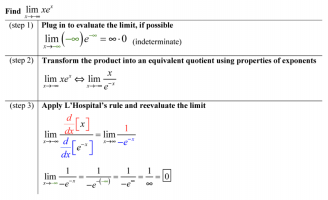from https://calcworkshop.com/derivatives/lhopitals-rule/

I then differentiated both to get

$(-1/4)/(2x-5)exp(x^2-5x+6)$
I thought I would have to differentiate again? but is the limit now determinate as $(-1/4)/((2x-5)exp(x^2-5x+6) = 0/infinity$
differentiating again I was following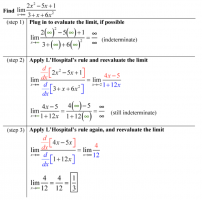In an attempt to prove directly that f(x) = 0 when x tends to infinity

Jomo

Elite Member
Since you changed the problem you need to see if you need to even use L'Hopital and whether or not you are allowed to even use it.
As x->oo, what is the numerator approaching?
As x->oo, what is the denominator approaching?

New member
Since you changed the problem you need to see if you need to even use L'Hopital and whether or not you are allowed to even use it.
As x->oo, what is the numerator approaching?
As x->oo, what is the denominator approaching?
I am still quite lost with this problem, I have never worked with limits before.

These are my current workings out, which I think support the use of L'Hopital since g'(x) does not = 0, both are differentiable, and the limits are indetermined?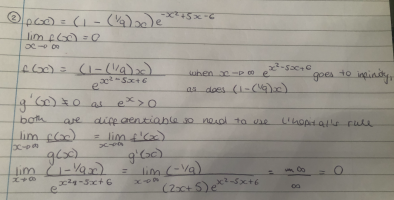Jomo

Elite Member
The very end say infinity/infinity = 0. Why do you think that infinity/infinity = 0? Also, why is -1/9 approaching infinity as x goes anywhere?

New member
Thank you for your reply, would it then be that as x goes to infinity -1/9 would go to zero, meaning it would be zero/infinity, and therefore zero?

Dr.Peterson

Elite Member
Thank you for your reply, would it then be that as x goes to infinity -1/9 would go to zero, meaning it would be zero/infinity, and therefore zero?
Not quite. -1/9 is a constant, and doesn't go anywhere! Its limit is -1/9.

So if you divide a negative constant by a function that goes to infinity, what is the limit?

New member
Not quite. -1/9 is a constant, and doesn't go anywhere! Its limit is -1/9.

So if you divide a negative constant by a function that goes to infinity, what is the limit?
Thank you, would it then also be 0?, I know from the question that f(x)=0 as lim x-> infinity

Dr.Peterson

Elite Member
Thank you, would it then also be 0?, I know from the question that f(x)=0 as lim x-> infinity
Yes, the limit is zero. It was only your work that was wrong.